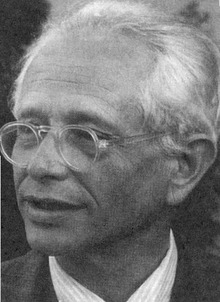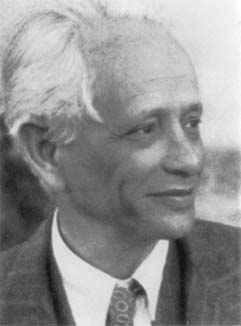# on Geometry, Topology, Dynamics, and Groups

### Fall 2011

 Date Speaker Title — click for abstract (if available) September 7 Patrick Reynolds University of Utah The boundary of Outer space This is a survey about the (usual) boundary of the Culler-Vogtmann Outer space. After briefly recalling the definition of Outer space, we will define a compactification and mention some results related to the boundary of this compactification. Topics will include: what is known about the minset for the action of $$Out(F_n)$$ on the boundary, the structure of very small actions of $$F_n$$ on $$\mathbb{R}$$-trees, and algebraic laminations. Analogies with the Thurston compactification of Teichmüller space will be emphasized. September 14 Patrick Reynolds University of Utah Indecomposable trees and arational algebraic laminations Associated to a tree $$T$$ in the boundary of Outer space is a symbolic dynamical system called the dual lamination of $$T$$, denoted $$L^2(T)$$. We characterize trees $$T$$ such that $$L^2(T)$$ is "minimal". Our techniques center around a two-part inductive procedure for studying $$L^2(T)$$. One part was known: it is a slight generalization of the Rips machine; the other part is new and is a generalization of the classical Rauzy induction. As an application of our techniques, we give a characterization of trees $$T$$ such that $$L^2(T)$$ is arational. Joint with T. Coulbois and A. Hilion. September 21 Jessica Purcell Brigham Young University The geometry of unknotting tunnels An unknotting tunnel is an arc in a 3-manifold M with torus boundary, such that the complement of the tunnel in M is a handlebody. Classically, one can "unknot" a knot or link by pulling its diagram along an unknotting tunnel. In 1995, Adams, and Sakuma and Weeks, asked three questions concerning the geometry of unknotting tunnels in a hyperbolic 3-manifold: Are they geodesic? Do they have bounded length? Are they canonical? While the answer to the first question is still open, we will describe fairly complete answers to all three questions in the case where M is created by a "generic" Dehn filling. As an application, there is an explicit family of knots in the 3-sphere whose tunnels are arbitrarily long. This is joint with Daryl Cooper and David Futer. September 28 Alden Walker California Institute of Technology Random rigidity in the free group (Joint work with Danny Calegari) We prove a rigidity theorem for the geometry of the unit ball in random subspaces of the scl norm (equivalently, the relative 2-dimensional Gromov norm) in $$B_1^H$$ of a free group. In a free group $$F$$ of rank $$k$$, a random word $$w$$ of length $$n$$ (conditioned to lie in $$[F,F]$$) has $\text{scl}(w) = \log \frac{(2k-1)n}{6 \log(n)} + O \left( \frac{n}{\log(n)} \right)$ with high probability, and the unit ball in a subspace spanned by $$d$$ random words of length $$O(n)$$ is $$C^0$$ close to a (suitably affinely scaled) octahedron. A conjectural generalization to hyperbolic groups and manifolds would show that the length of a random geodesic in a hyperbolic manifold can be recovered from the bounded cohomology of the fundamental group. October 5 Mladen Bestvina University if Utah Geometry of Integral Binary Hermitian Forms We generalize Conway's approach to integral binary quadratic forms on $$\mathbb Q$$ to study integral binary hermitian forms on quadratic imaginary extensions of $$\mathbb Q$$. In Conway's case, an indefinite form that doesn't represent $$0$$ determines a line ("river") in the spine T associated with $$\text{SL}(2, \mathbb Z)$$ in the hyperbolic plane. In our generalization, such a form determines a plane ("ocean") in Mendoza's spine associated with the corresponding Bianchi group $$\text{SL}(2,A)$$ in hyperbolic 3-space. This is joint work with Gordan Savin. November 2 Jing Tao University of Utah T.B.A. November 9 Sebastian Hensel University of Bonn Sphere systems and the geometry of Out(F_n) By a theorem of Laudenbach, the outer automorphism group $$\mathrm{Out}(\mathbb{F}_n)$$ of a free group is a cofinite quotient of the mapping class group of a suitable 3-manifold $$M_n$$. This point of view allows to study the geometry of $$\mathrm{Out}(\mathbb{F}_n)$$ with methods inspired from surface mapping class groups. In this talk, we present an application of this strategy: we show that the natural inclusion of the mapping class group of a surface of genus $$g$$ with one puncture into $$\mathrm{Out}(\mathbb{F}_{2g})$$ is undistorted. The results are joint work with Ursula Hamenstädt. November 16 Jorg Thuswaldner Leoben/BYU Rational self-affine tiles In this talk I study self-affine tiles that are defined using rational matrices. These tiles are subsets of the direct product of $$\mathbb{R}^n$$ with a number of $$p$$-adic completions of a number field K. We study tiling properties of these tiles and discuss their relation to numeration.

Current Seminar          Archive of past talks
Max Dehn Seminar is organized by Mladen Bestvina, Ken Bromberg, Patrick Reynolds,
Jing Tao, Domingo Toledo, and Kevin Wortman.

This web page is maintained by Patrick Reynolds and Jing Tao.